# CBSE Sample Papers for Class 10 Maths Paper 11

These Sample papers are part of CBSE Sample Papers for Class 10 Maths. Here we have given CBSE Sample Papers for Class 10 Maths Paper 11.

## CBSE Sample Papers for Class 10 Maths Paper 11

 Board CBSE Class X Subject Maths Sample Paper Set Paper 10 Category CBSE Sample Papers

Students who are going to appear for CBSE Class 10 Examinations are advised to practice the CBSE sample papers given here which is designed as per the latest Syllabus and marking scheme as prescribed by the CBSE is given here. Paper 11 of Solved CBSE Sample Paper for Class 10 Maths is given below with free pdf download solutions.

Time allowed: 3 Hours
Maximum Marks: 80

General Instructions

• All questions are compulsory.
• The question paper consists of 30 questions divided into four sections A, B, C and D.
• Section A contains 6 questions of 1 mark each. Section B contains 6 questions of 2 marks each. Section C contains 10 questions of 3 marks each. Section D contains 8 questions of 4 marks each.
• There is no overall choice. However, an internal choice has been provided in four questions of 3 marks each and three questions of 4 marks each. You have to attempt only one of the alternatives in all such questions.
• Use of calculators is not permitted.

Section – A

Question 1.
Write whether the rational number $\frac { 7 }{ 75 }$ will have a terminating decimal expansion or a non-terminating repeating decimal expansion.

Question 2.
Find the value (s) of k, if the quadratic equation 3x2 – k √3x + 4 = 0 has equal roots.

Question 3.
Find the eleventh term from the last term of the AP: 27, 23, 19,… ,- 65.

How do you round 214.0822 to the nearest tenth? … Hint: We are given a decimal number.

Question 4.
Find the coordinates of the point on y- axis which is nearest to the point (-2, 5).

Question 5.
In given figure, ST || RQ, PS = 3 cm and SR = 4 cm. Find the ratio of the area of ∆ PST to the area of ∆ PRQ.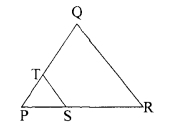Question 6.
If cos A = $\frac { 2 }{ 5 }$ , find the value of 4 + 4 tan2 A.

Section – B

Question 7.
If two positive integers p and q are written as p = a2 b3 and q = a3 b; a, b are prime numbers, then verily: LCM (p, q) × HCF (p, q) = pq

Question 8.
The sum of first n terms of an AP is given by Sn = 2n2 + 3n. Find the sixteenth term of the A.P.

Question 9.
Find the value (s) of k for which the pair of linear equations kx + y = k2 and x + ky = 1 have infinitely many solutions.

Question 10.
If ( 1, $\frac { p }{ 3 }$ ) is the mid- point of the line segment joining the points (2,0) and ( 0, $\frac { 2 }{ 9 }$ ) then show that the line 5x + 3y + 2 = 0 passes through the point (-1 , 3p)

Question 11.
A box contains cards numbered 11 to 123. A card is drawn at random from the box. Find the probability that the number on the drawn card is

1. a square number
2. a multiple of 7.

To convert 10 11/16 to decimal you can use the long division method explained in our article fraction to decimal, which you can find in the header menu.

Question 12.
A box contains 12 balls of which some are red in colour. If 6 more red balls are put in the box and a ball is drawn at random, the probability of drawing a red ball doubles than what it was before. Find the number of red balls in the bag.

Section – C

Question 13.
Show that exactly one of the numbers n, n + 2 or n + 4 is divisible by 3.

Question 14.
Find all the zeroes of the polynomial 3x4 + 6x3 – 2x2 – 10x – 5 if two of its zeroes are √ $\frac { 5 }{ 3 }$ and – √ $\frac { 5 }{ 3 }$

Question 15.
Seven times a two digit number is equal to four times the number obtained by reversing the order of its digits. Ifthe difference ofthe digits is 3, determine the number.

Question 16.
In what ratio does the x-axis divide the line segment joining the points (-4,-6) and (-1, 7)? Find the co-ordinates of the point of division.
OR
The points A (4, -2), B (7,2), C (0,9) and D (-3,5) form a parallelogram. Find the length of the altitude of the parallelogram on the base AB.

Question 17.
In given figure ∠1 = ∠2 and ∆NSQ = ∆MTR, then prove that ∆ PTS ~ PRQ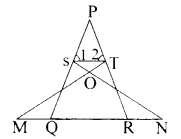OR
In an equilateral triangle ABC, D is a point on the side BC such that BD = $\frac { 1 }{ 3 }$ BC. Prove that 9 AD2 = 7 AB2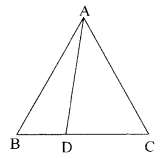Question 18.
In given figure XY and X’ Y’ are two parallel tangents to a circle with centre O and another tangent AB with point of contact C intersecting XY at A and X’ Y’ at B. Prove that ∠ AOB = 90°.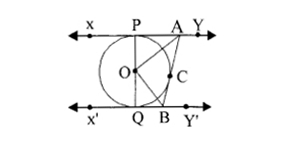Question 19.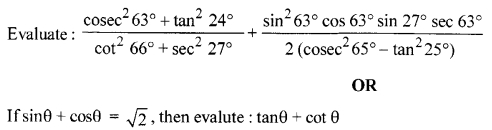Question 20.
In given figure ABPC is a quadrant of a circle ofradius 14 cm and a semicircle is drawn with BC as diameter. Find the area of the shaded region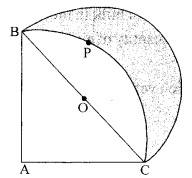Question 21.
Water in a canal, 6 m wide and 1.5m deep, is flowing with a speed of 10 km/h. How much area will it irrigate in 30 minutes, if 8 cm of standing water is needed?
OR
A cone of maximum size is carved out from a cube of edge 14 cm. Find the surface area of the remaining solid after the cone is carved out.

Question 22.
Find the mode of the following distribution of marks obtained by the students in an examination :

 Marks obtained 0-20 20-40 40-60 60-80 80-100 Number of students 15 18 21 29 17

Given the mean of the above distribution is 53, using empirical relationship estimate the value of its median.

Question 23.
A train travelling at a uniform speed for 360 km would have taken 48 minutes less to travel the same distance if its speed were 5 km/hour more. Find the original speed of the train.
OR
Check whether the equation 5x2 – 6x – 2 = 0 has real roots and if it has, find them by the method of completing the square. Also verify that roots obtained satisfy the given equation.

Question 24.
An AP consists of 3 7 terms. The sum of the three middle most terms is 225 and the sum of the last three terms is 429. Find the AP.

Question 25.
Show that in a right triangle, the square of the hypotenuse is equal to the sum of the squares of the other two sides.
OR
Prove that the ratio of the areas of two similar triangles is equal to the ratio of the squares of their corresponding sides.

Question 26.
Draw a triangle ABC with side BC = 7 cm, ∠B=45°, ∠A= 105°. 1 Then, construct a triangle whose sides are $\frac { 4 }{ 3 }$ times the corresponding sides of ∆ABC.

Question 27.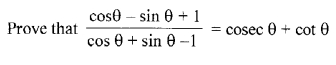Question 28.
The angles of depression of the top and bottom of a building 50 metres high as observed from the top of a tower are 30° and 60°, respectively. Find the height of the tower and also the horizontal distance between the building and the tower.

Question 29.
Two dairy owners A and B sell flavoured milk filled to capacity in mugs of negligible thickness, which are cylindrical in shape with a raised hemispherical bottom. The mugs are 14 cm high and have diameter of 7 cm as shown in given figure. Both A and B sell flavoured milk at the rate of ₹ 80 per litre. The dairy owner A uses the formula nrh to find the volume of milk in the mug and charges ₹ 43.12 for it. The dairy owner B is of the view that the price of actual quantity of milk should be charged. What according to him should be the price of one mug of milk? Which value is exhibited by the dairy owner B ? [ use π = $\frac { 22 }{ 7 }$ )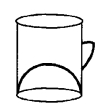Question 30.
The following distribution shows the daily pocket allowance of children of a locality. The mean pocket allowance is ₹ 18. Find the missing frequency k.

 Daily pocket allowance (in ₹) 11-13 13-15 15-17 17-19 19-21 21-23 23-25 Number of children 3 6 9 13 k 5 4

The following frequency distribution shows the distance (in metres) thrown by 68 students in a Javelin throw competition.

 Distance (in m) 0-10 10-20 20-30 30-40 40-50 50-60 60-70 Number of students 4 5 13 20 14 8 4

Draw a less than type O give for the given data and find the median distance thrown using this curve.

Solutions

Solution 1.
Non terminating repeating decimal expansion.

Solution 2.
k = ± 4

Solution 3.
a11 = -25

Solution 4.
(0,5)

Solution 5.
9 : 49

Solution 6.
4 + tan2 A = 4 sec2 A = 4 ( $\frac { 5 }{ 2 }$ ) 2 = 25

Solution 7.
LCM (p, q) = a3 b3
HC,F (p, q) = a2 b
LCM (p, q) × HCF (p, q) = a5 b4 = (a2 b3)
(a3 b) = pq

Solution 8.
Sn = 2n2 + 3n
S1 = 5 = a1
S2 = a1 + a2 = 14 ⇒ a2 = 9
d= a2 – a1 = 4
a16 = a1 + 15d = 5+ 15(4) = 65

Solution 9.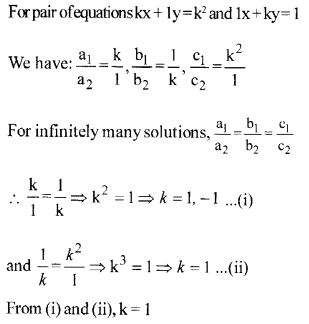Solution 10.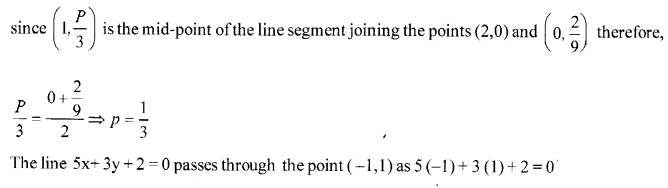Solution 11.

1. P (square number) = $\frac { 8 }{ 113 }$
2. P (multiple of 7) = $\frac { 16 }{ 113 }$

Solution 12.
Let number of red balls be = x
∴ P (red ball) = $\frac { x }{ 12 }$
If 6 more red balls are added: The number of red balls = x + 6 P (red ball) = $\frac { x+6 }{ 18 }$
Since, $\frac { x+6 }{ 18 }$ = 2 ( $\frac { x }{ 12 }$ ) ⇒ x = 3
There are 3 red balls in the bag.

Solution 13.
Let n = 3k, 3k + 1 or 3k+ 2.
(i) When n = 3k: n is divisible by 3.
n + 2 = 3k + 2 ⇒ n + 2 is not divisible by 3.
n + 4 = 3k + 4 = 3 (k + 1)+ 1 ⇒ n + 4 is not divisible by 3.

(ii) When n = 3k + 1: n is not divisible by 3.
n + 2 = (3k + 1) + 2 = 3k+ 3 = 3 (k+ 1) ⇒ n + 2 is divisible by 3.
n + 4 = (3k+ l) + 4 = 3k + 5 = 3(k+ 1) + 2 ⇒ n + 4isnotdivisibleby3.

(iii) When n = 3k + 2: n is not divisible by 3.
n + 2 = (3k+2) + 2 = 3k + 4 = 3(k+ 1)+ 1 ⇒ n + 2 is not divisible by 3.
n + 4 = (3k + 2)+ 4 = 3k+ 6 = 3(k + 2) ⇒ n + 4 is divisible by 3.
Hence exactly one of the numbers n, n + 2 or n + 4 is divisible by 3.

Solution 14.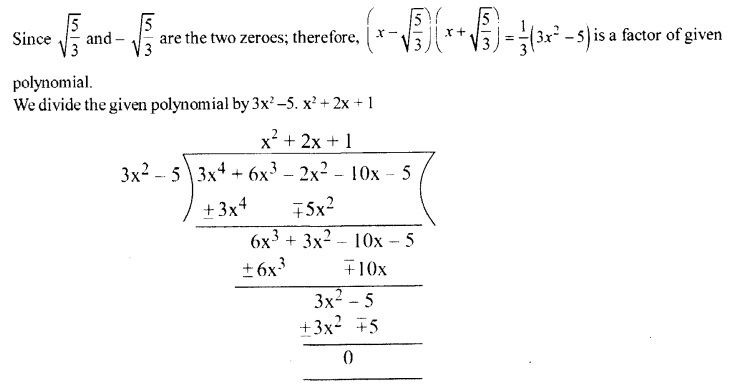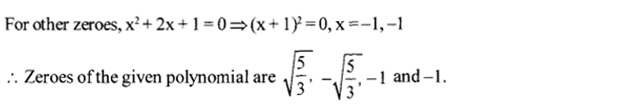Solution 15.
Let the ten’s and the units digit be y and x respectively.
So,the number is 10 y + x.
The number when digits are reversed is 10 x + y.
Now, 7(10y+ x) = 4(10x + y) ⇒ 2y = x …( 1)
Also x – y3 …(ii)
Solving (1) and (2),we get y = 3 and x = 6.
Hence the number is 36.

Solution 16.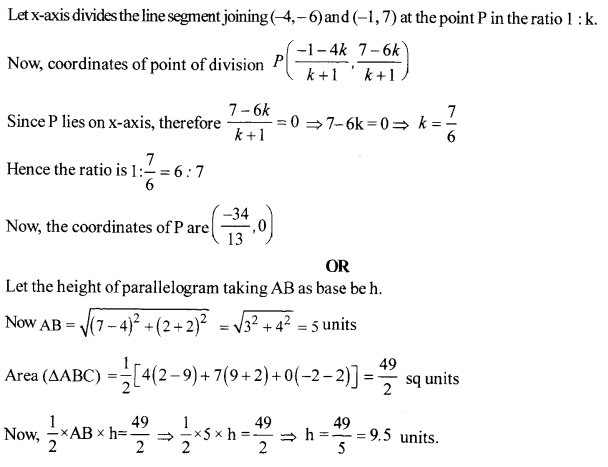Solution 17.
∠SQN = ∠TRM (CPCT as NSQ ≅ MTR)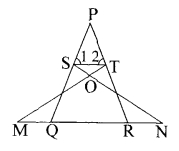Since, ∠P + ∠1 + ∠2 = ∠P + ∠PQR + ∠PRQ (Angle sum property)
⇒ ∠1 + ∠2 = ∠PQR + ∠PRQ
⇒ 2 ∠1 = 2 ∠PQR (as ∠1 = ∠2 and ∠PQR= ∠PRQ) ⇒ ∠1 = ∠PQR
Also ∠2 = ∠PRQ and ∠SPT = ∠QPR (common) ∆PTS ~ ∆PRQ (By AAA similarity criterion)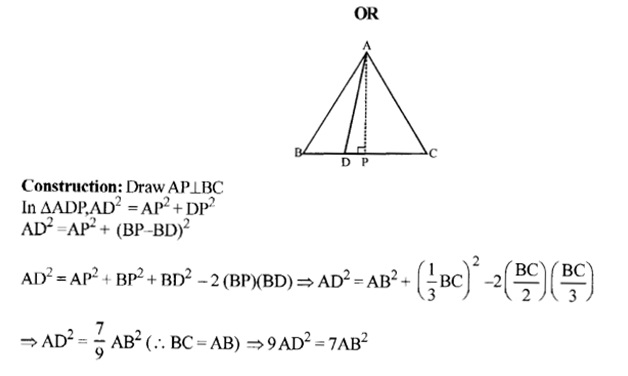Solution 18.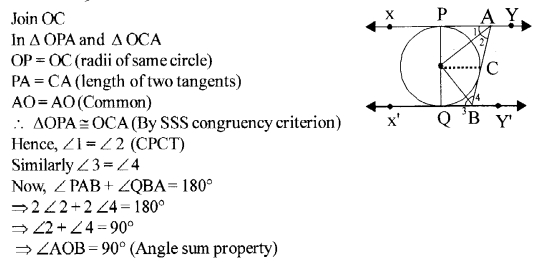Solution 19.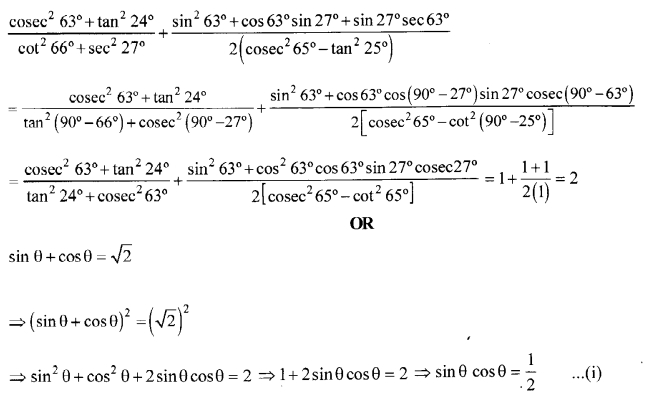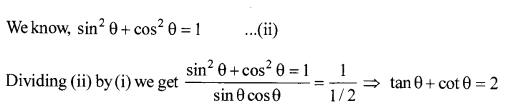Solution 20.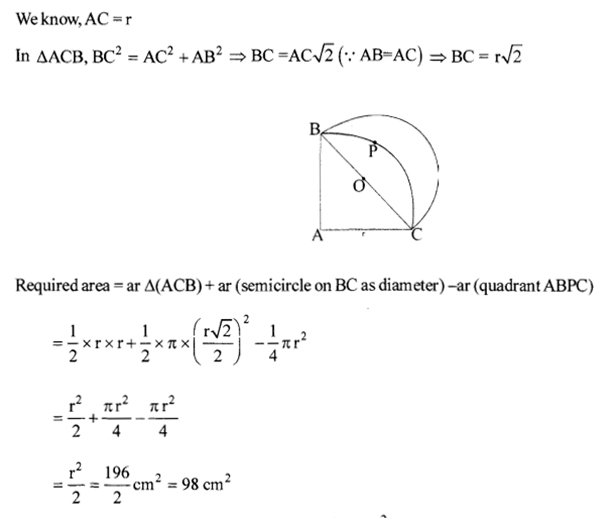Solution 21.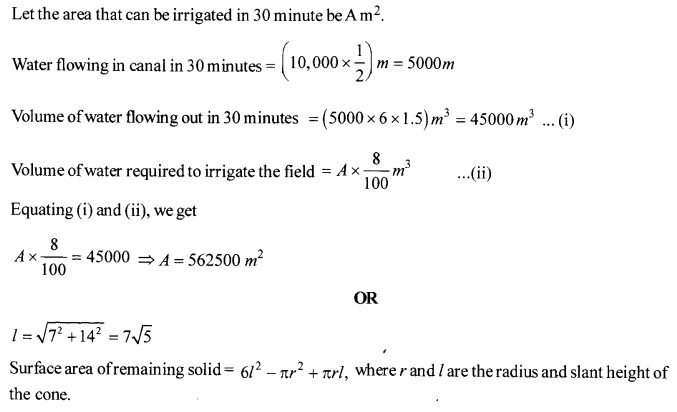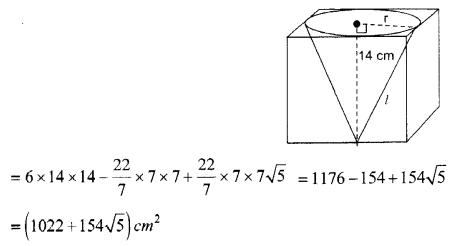Solution 22.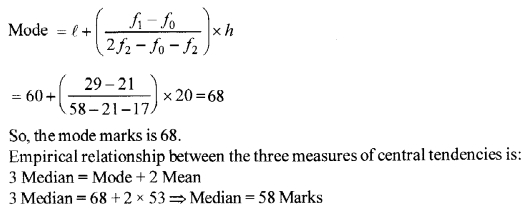Solution 23.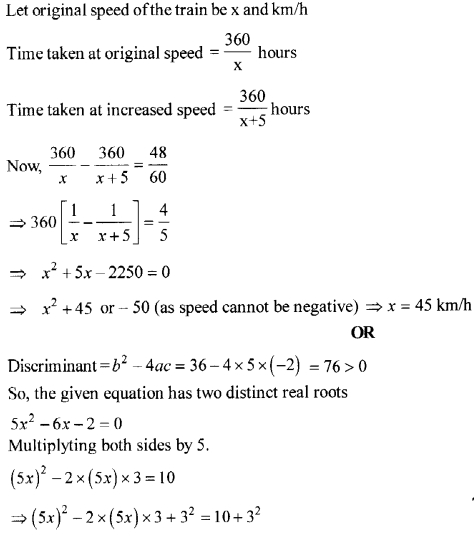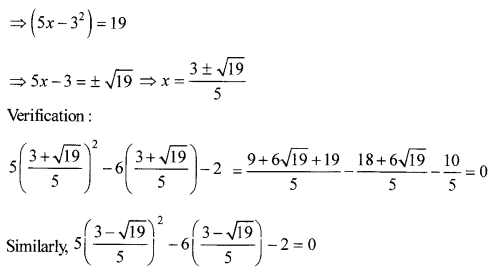Solution 24.
Let the three middle most terms of the AP be a – d, a, a + d
We have, (a – d) +a + (a + d) = 225
⇒ 3 a = 225 ⇒ a = 75
Now, the AP is a – 18d,…,a – 2d, a – d, a, a + d,a + 2d,…,a + 18d
Sum of last three terms :
(a + 18d) + (a + 17d) + (a + 16d) = 429
⇒ 3a + 51 d = 429 ⇒ (a + 17d) = 143
⇒ 75 + 17d = 143 ⇒ d = 4
Now, first term = a – 18d = 75 – 18(4) = 3
∴ The AP is 3,7,11,…, 147.

Solution 25.
Given : A right triangle ABC right angled at B.
To prove : AC2 = AB2 + BC2
Construction : Draw BD ⊥ AC
Proof : In ∆ ADB and ∆ ABC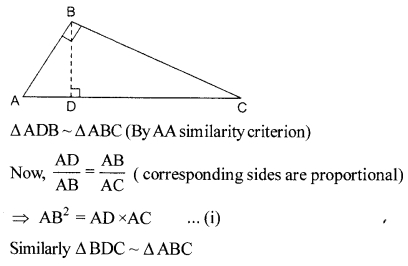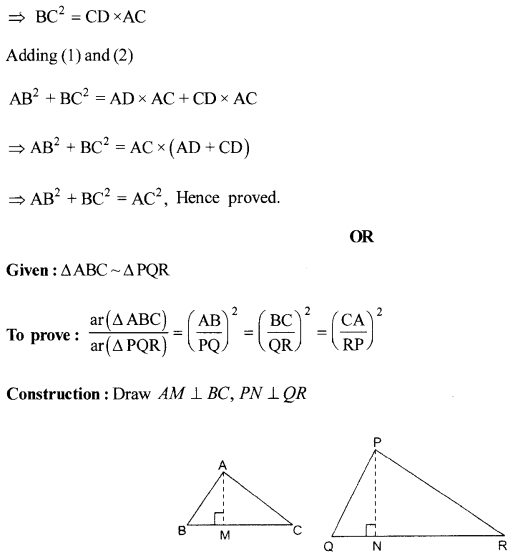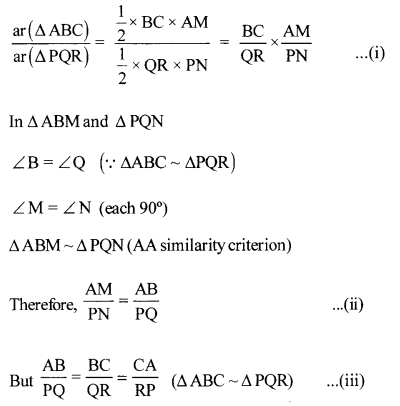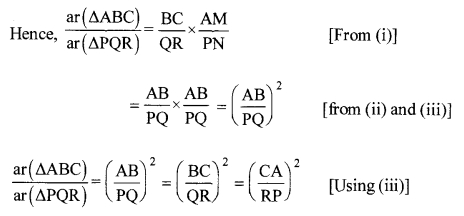Solution 26.
Draw ∆ABC in which BC = 7 cm, ∠B = 45°, ∠A = 105° and hence ∠C = 30°.
Construction of similar triangle A’ BC’ as shown below.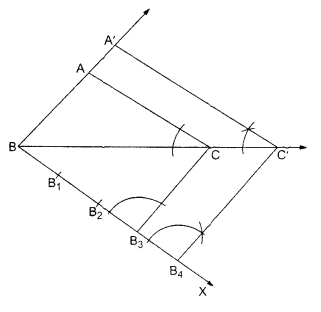Solution 27.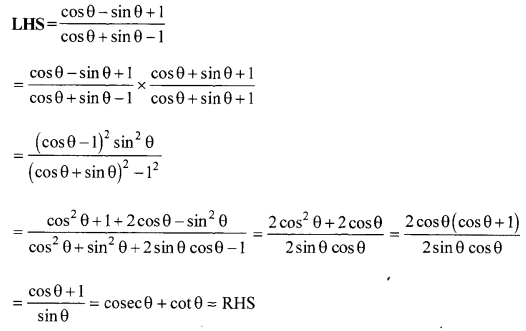Solution 28.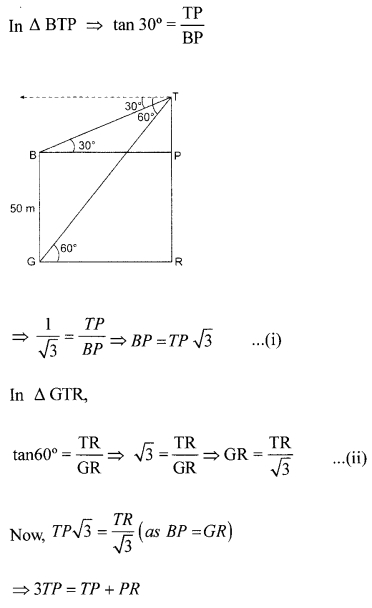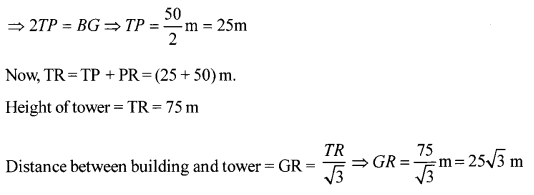Solution 29.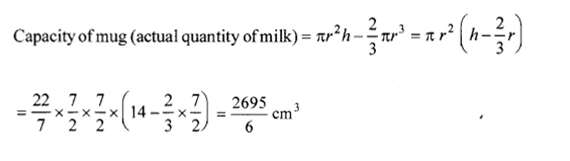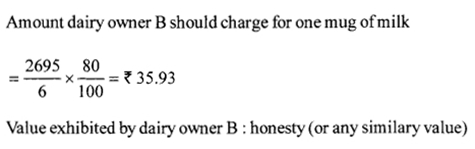Solution 30.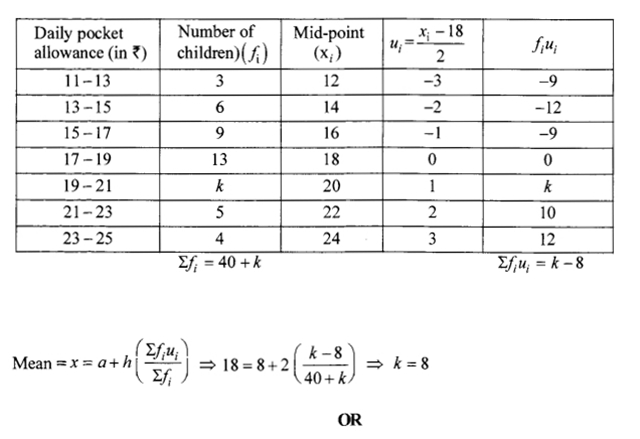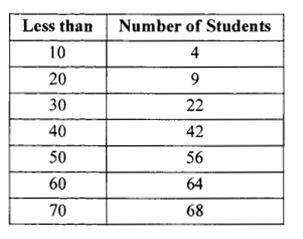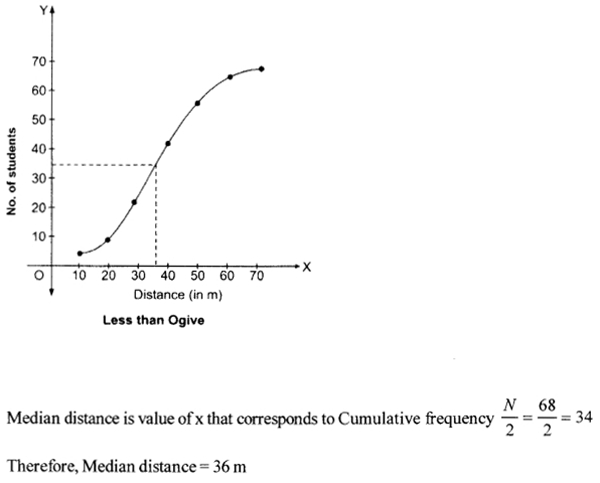We hope the CBSE Sample Papers for Class 10 Maths paper 11 help you. If you have any query regarding CBSE Sample Papers for Class 10 Maths paper 11, drop a comment below and we will get back to you at the earliest.